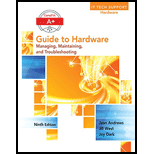Chapter 5, Problem 1TC### A+ Guide to Hardware (Standalone B...

9th Edition
Jean Andrews
ISBN: 9781305266452

#### Solutions

Chapter
Section### A+ Guide to Hardware (Standalone B...

9th Edition
Jean Andrews
ISBN: 9781305266452
Textbook Problem
1 views

# How much power is consumed by a load drawing 5 A with 120 V across it?

Program Explanation

Given: A load with 5 A current and 120 V voltage.

To find:The power consumed by the load which is using 5 A of current and 120 V of voltage.

Solution:

The formula to find the power consumed is:

Watt=Voltage×Amp=120×5=600 watts

Hence, the power consumed is 600 watts.

#### The Solution to Your Study Problems

Bartleby provides explanations to thousands of textbook problems written by our experts, many with advanced degrees!

Get Started